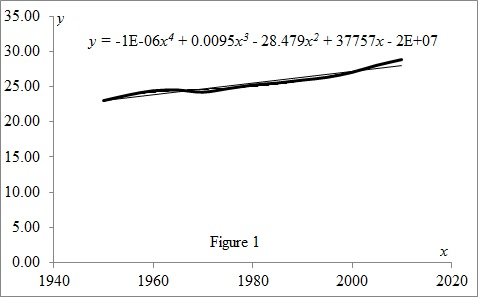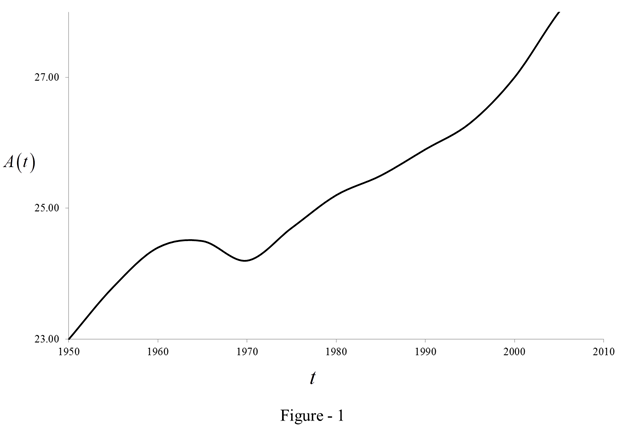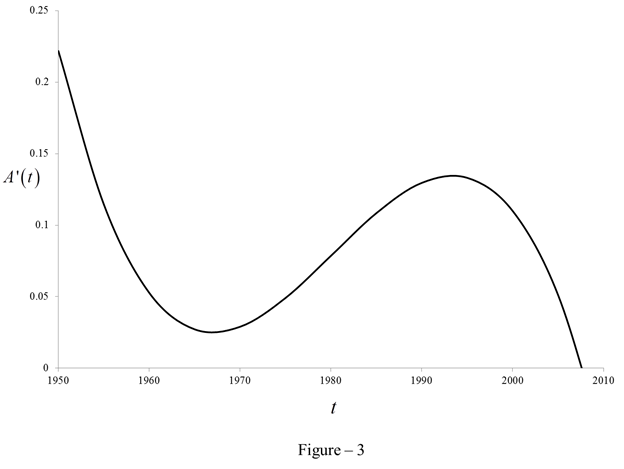# To model the given data with a fourth-degree polynomial.### Single Variable Calculus: Concepts...

4th Edition
James Stewart
Publisher: Cengage Learning
ISBN: 9781337687805### Single Variable Calculus: Concepts...

4th Edition
James Stewart
Publisher: Cengage Learning
ISBN: 9781337687805

#### Solutions

Chapter 3.8, Problem 26E

(a)

To determine

## To find: To model the given data with a fourth-degree polynomial.

Expert Solution

The fourth-degree polynomial is y=1.2×106t4+9545.853t328.48t2+37757.11t1.88×107.

### Explanation of Solution

Given:

The average age of first marriage of Japanese women has varied since 1950 is shown in table (1).

 t A(t) 1950 23.00 1955 23.80 1960 24.40 1965 24.50 1970 24.20 1975 24.70 1980 25.20 1985 25.50 1990 25.90 1995 26.30 2000 27.00 2005 28.00

Calculation:

Model the given data with a fourth-degree polynomial.

A(t)=at4+bt3+ct2+dt+e (1)

Plot the graph between t and A(t) using table (1) as shown in figure (1).Refer the figure (1).

The fourth-degree polynomial for the curve is as below.

A(t)=1.2×106t4+0.0095t328.48t2+37757.11t1.88×107 (2)

Equate the equation (1) with equation (2).

at4+bt3+ct2+dt+e=1.2×106t4+0.0095t328.48t2+37757.11t1.88×107

Compare the above equation.

a=1.2×106, b=0.0095, c=28.48, d=37757.11 and e=1.88×107.

(b)

To determine

### To find: The model for A'(t) by using part (a).

Expert Solution

The value of A'(t) is 0.000005t3+28637.55t256.96t+37757.11.

### Explanation of Solution

Calculate the value of A'(t).

Differentiate equation (1) with respect to t.

A'(t)=4at3+3bt2+2ct+d (3)

Substitute 1.2×106 for the expression a, 9545.853 for the expression b, 28.48 for the expression c and 37757.11 for the expression d.

A'(t)=4(1.2×106)t3+3(0.0095)t2+2(28.48)t+37757.11

A'(t)=0.000005t3+0.0285t256.96t+37757.11 (4)

Thus, the value of A'(t) is 0.000005t3+0.0285t256.96t+37757.11.

(c)

To determine

### To estimate: The rate of change of marriage age for women in 1990.

Expert Solution

The rate of change of marriage age for women in 1990 is 2,133.44.

### Explanation of Solution

Compute the rate of change of marriage age for women in 1990 using the formula below.

A'(t)=0.000005t3+0.0285t256.96t+37757.11

Substitute 1990 for t.

A'(t)=0.000005×1,9903+0.0285×1,990256.96×1,990+37,757.11=2,133.44

(d)

To determine

Expert Solution

### Explanation of Solution

Plot the graph between t and A(t) as shown in Figure (2).Calculate the difference between the year 1950 and 1955.

Difference=19501945=5

Substitute the value 5 for the expression t in the equation (2).

A'(t)=0.000005(5)3+28637.55(5)256.96(5)+37757.11=0.114614

Similarly, calculate the value of A'(t) for the following values of t in table (1).

Tabulate the value of A'(t) as shown in table (2).

 Difference A'(t) 0 0.221834 5.0 0.114614 10 0.052734 15.0 0.027194 20 0.028994 25.0 0.049134 30 0.078614 35.0 0.108434 40 0.129594 45.0 0.133094 50 0.109934 55.0 0.051114 60 -0.052366

Plot the graph between t and A'(t) using the table (2) as shown in Figure (3).### Have a homework question?

Subscribe to bartleby learn! Ask subject matter experts 30 homework questions each month. Plus, you’ll have access to millions of step-by-step textbook answers!